# 了解 DAX 函数中 LASTDATE 和 MAX 之间的区别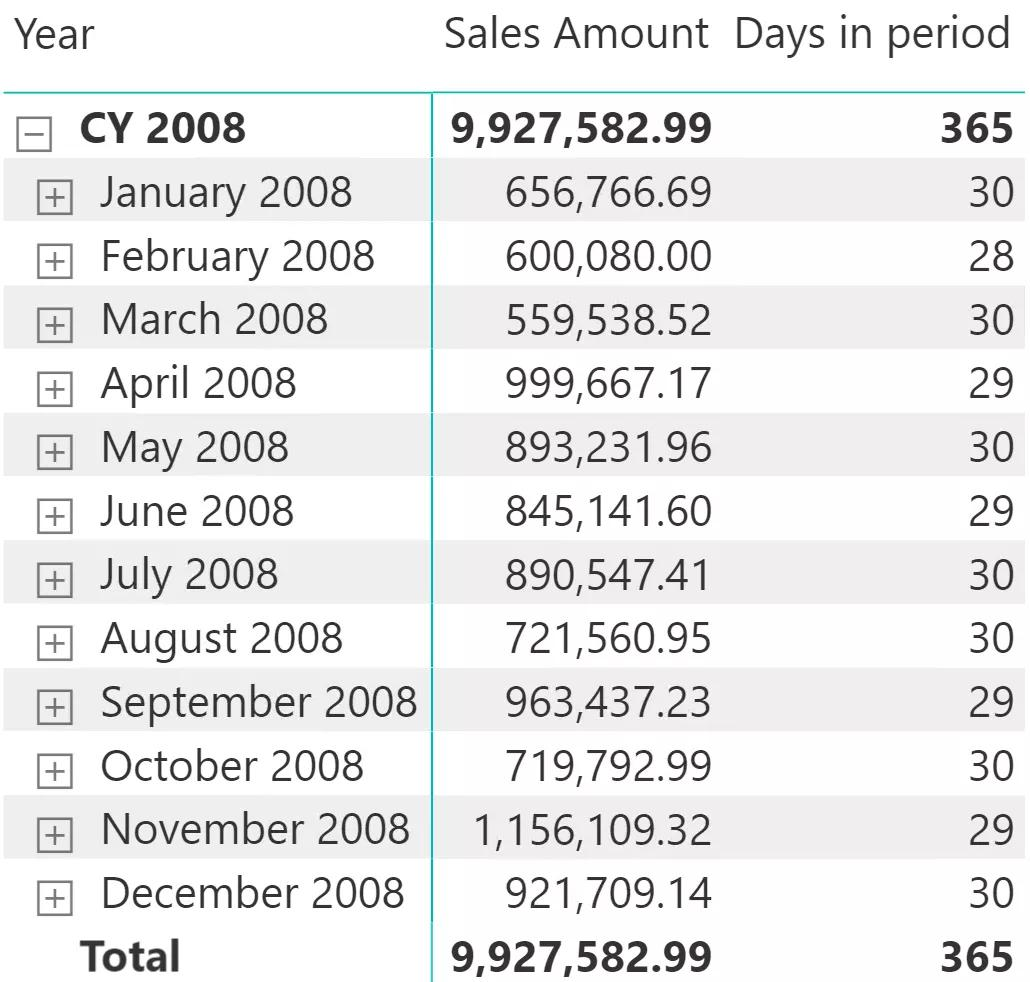Days in period :=INT ( LASTDATE ( 'Date'[Date] ) - FIRSTDATE ( 'Date'[Date] ) )

SalesOfLastDay =CALCULATE ( [Sales Amount], LASTDATE ( 'Date'[Date] ))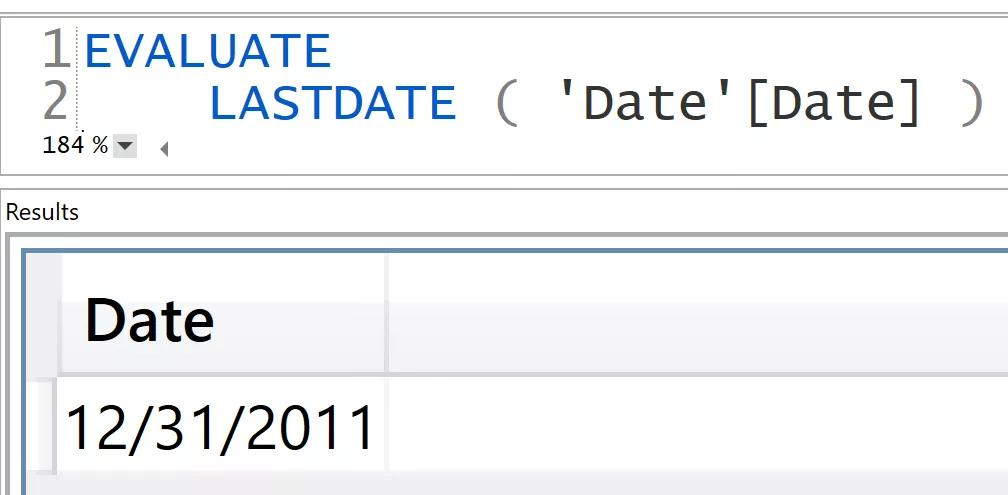Days in period :=INT ( LASTDATE ( 'Date'[Date] ) - FIRSTDATE ( 'Date'[Date] ) )

Days in period MIN MAX :=INT ( MAX ( 'Date'[Date] ) - MIN ( 'Date'[Date] ) )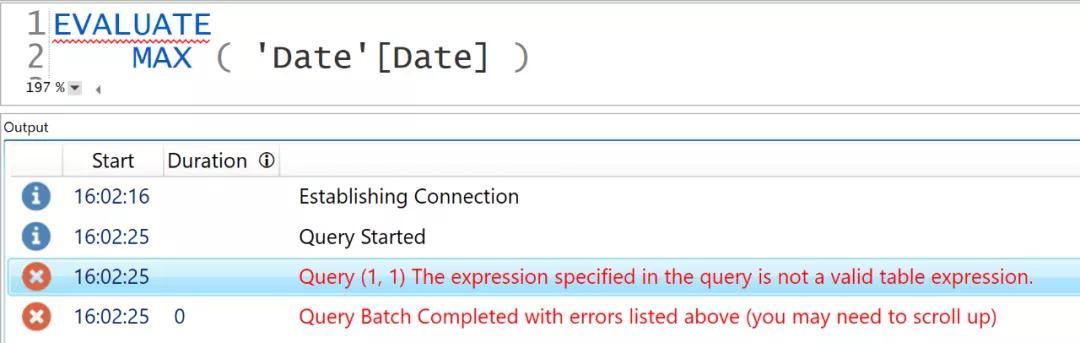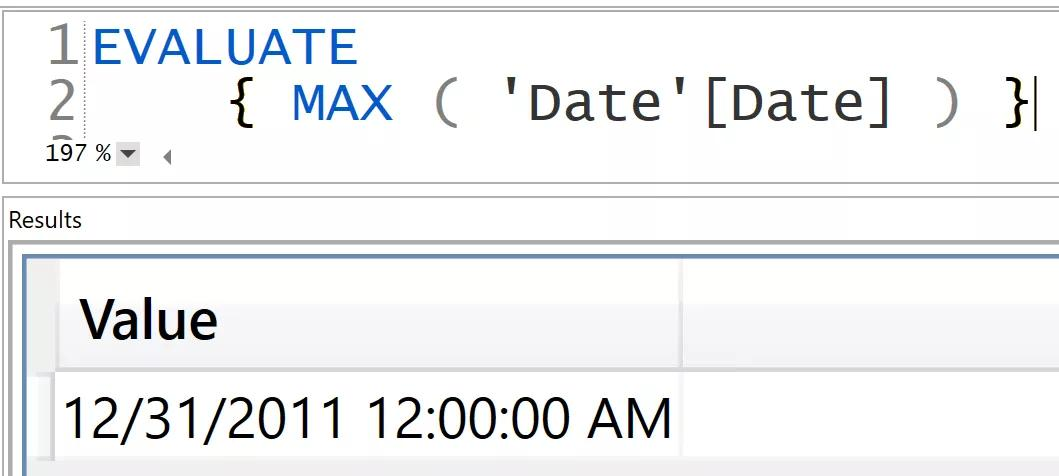---- This version uses FIRSTDATE and LASTDATE

--

EVALUATE

SUMMARIZECOLUMNS (

'Date'[Year Month],

"Days in period",

[Days in period]

)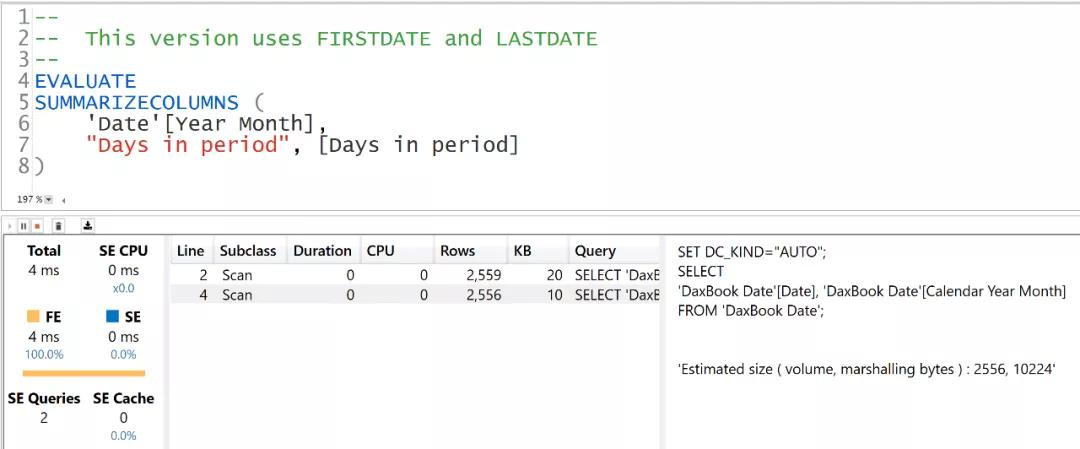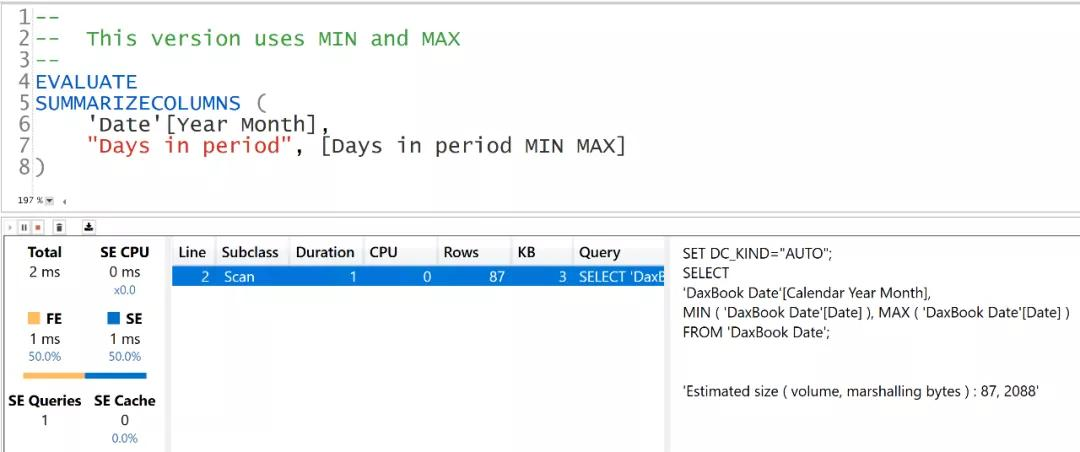Power Pivot工坊